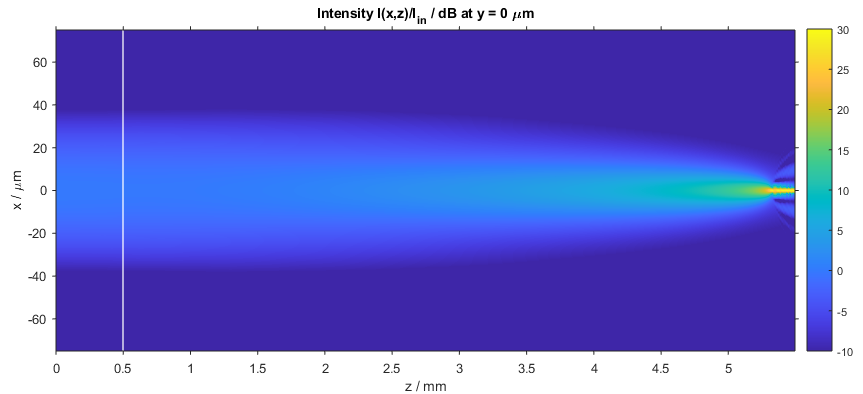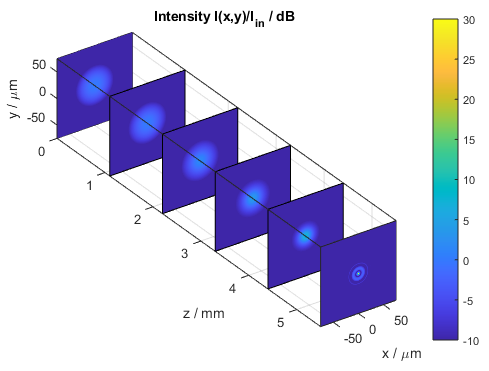﻿ Optical simulation of a nonlinear Kerr medium - BeamLab

# Nonlinear Self-focusing

This BeamLab demo shows a high-power Gaussian beam incident on a block of fused silica where it then converges to a small spot due to the self-focusing effect by taking into account the nonlinear change of the intensity-dependent refractive index.

BeamLab demo: selffocusing.m

The following video shows how the intensity distribution changes along the propagation distance z. The left figure shows the two-dimensional intensity distribution I(x,y) normalized to the input intensity and displayed on linear scale. The right figure shows a three-dimensional view of the intensity dependent nonlinear refractive index.

Video of intensity profile (left) and refractive index (right)

After the calculation has finished, the intensity distribution as a function of propagation distance z is plotted. The figure below depicts the intensity distribution I(x,z) at y = 0 µm. The white line indicates the start of the block of fused silica.Intensity distribution of the self-focusing Gaussian beam (xz slice)

The following figure shows a compact three-dimensional view of stacked plots, each portraying the intensity distribution I(x,y) at different distances z.Intensity distribution of the self-focusing Gaussian beam (stacked xy slices)